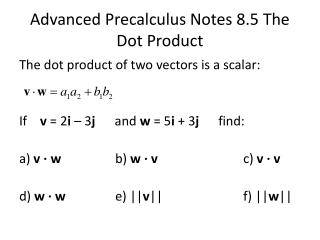DownloadDownload PresentationAdvanced Precalculus Notes 8.5 The Dot Product

# Advanced Precalculus Notes 8.5 The Dot Product

Download Presentation## Advanced Precalculus Notes 8.5 The Dot Product

- - - - - - - - - - - - - - - - - - - - - - - - - - - E N D - - - - - - - - - - - - - - - - - - - - - - - - - - -
##### Presentation Transcript

1. Advanced Precalculus Notes 8.5 The Dot Product The dot product of two vectors is a scalar: If v = 2i – 3j and w = 5i + 3j find: a) v ∙ w b) w ∙ v c) v ∙ v d) w ∙ w e) ||v|| f) ||w||

2. Properties of dot products: Commutative Property: u ∙ v = v ∙ u Distributive Property: u ∙ (v + w) = u ∙ v + u ∙ w v ∙ v = ||v||2 0 ∙ v = 0 Angle between Vectors: Find the angle between u = 4i -3j and v = 2i + 5j

3. A Boeing 737 aircraft maintains a constant airspeed of 500 mph due South. The velocity of the jet stream is 80 mph in a northeasterly direction. Find the actual speed and direction of the aircraft relative to the ground.

4. Parallel Vectors: if = 1 or -1, the vectors are parallel. Orthogonal Vectors: If the dot product is zero, the vectors are orthogonal (perpendicular). v = 3i – j w = 6i – 2ju = 2i – jz = 3i + 6j a) Are vectors v and w parallel, orthogonal of neither? b) Are vectors u and z parallel, orthogonal or neither?

5. Vector projection of v onto w: Find the vector projection of v = i +3j onto w = i + j

6. Work: work W done by a constant force F is: W = (magnitude of force)(distance) = An object is pulled with a force of 50 pounds. How much work is done in moving the object 100 feet if the handle makes an angle or 30º with the ground?

7. Assignment: page 626: 1 – 7, 12, 16, 17, 19, 21, 25, 27, 29, 32, 35